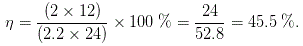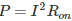# MCQs on Analog Electronics

##### Page 4 of 33. Go to page 1 2 3 4 5 6 7 8 9 10 11 12 13 14 15 16 17 18 19 20 21 22 23 24 25 26 27 28 29 30 31 32 33
01․ The majority carriers in the emitter of a PNP transistor are
holes.
free electrons.
trivalent atoms.
pentavalent atoms.

In a PNP transistor, the majority current carriers are holes. In an NPN transistor, the majority current carriers are electrons.

02․ An Op-Amp comparator circuit employs
no feedback.
+ve feedback.
-ve feedback.
both B and C.

Op-Amp comparator circuit is used to compare the difference between voltage level of two inputs (inverting and non-inverting) and also determines which one is greater. It requires no feedback circuit.

03․ In n type semiconductor elements of which group of periodic table is added as dopant
group 5.
group 2.
group 3.
group 4.

With group 5 elements like phosphorous, arsenic, antimony are added to Ge or Si crystal which produces free electrons. Group 5 elements has 5 electrons in their outermost shell. Four of them makes co-valance bond and remaining electron acts as charge carriers.

04․ Darlington connection is achieved in 2 transistors by connecting
both emitter.
both collector.
both base.
grounding both collector.

In darlington pair or connection 2 transistor's collectors are connected and emitter of 1st transistor is connected to the base of 2nd. This provides a three terminal device that in actual operation can be regarded as 2 cascaded emitter followers.

05․ A zener diode voltage regulator has load requirement of 12 V and 2 Amp. The zener diode's minimum current requirement is 0.2 A. The minimum voltage at input is 24 V. What is maximum efficiency of circuit?
34.3 %.
45.5 %.
52.8 %.
66.3 %.06․ The current gain of a pnp transistor is
the negative of the npn current gain.
the collector current divided by the emitter current.
near zero.
the ratio of collector current to emitter current.

Typically for a pnp or npn transistor, the current gain (α) is the ratio of the DC collector current to the DC emitter current. It is near to unity.

07․ A 3 - phase diode bridge rectifier is fed from a 400 V rms, 50 Hz, 3 phase AC source. If the load is purely resistive, then peak instantaneous output voltage is equal to
400√ 2 V.
400 V.
400√ (2/3) V.
400 / √ 3 V.

As the load is resistive the peak instantaneous output voltage Vm = 400√ 2 V.

08․ The conduction loss verses device current characteristics of power MOSFET in best approximately by
a parabola.
an exponentially decaying function.
a rectangular hyperbola.
a straight line.

I = Device current, Ron = on state resistance of power MOSFET, hence conduction loss. Therefore conduction loss verses device current characteristics is approximately a parabola.

09․ Which of the following cannot actually move?
Ions
Holes
Electrons
All the above

It is holes exactly because these are created by movement of deficiency electrons from one atom to another.

10․ Which material behave like perfect insulators at low temperatures & conductor at higher temperature ?
Si
Ge
GaAs
All the above

The materials are termed as semiconductor which behave like perfect insulators at low temperatures & at higher temperatures they behave like good conductors. At 0K semiconductor behaves like an insulator and when temperature increases it starts conducting.

<<<23456>>>